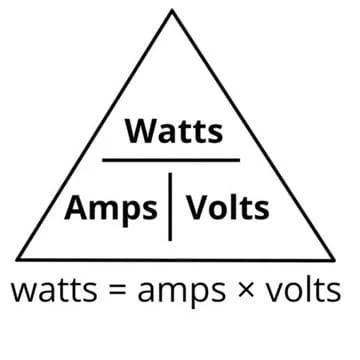top of page
Search

# Volts vs. Watts vs. Amps

Connecting your new equipment to the wrong power supply prevents its efficient operation, and it may even damage it.

Voltage, amperage, and wattage are all related elements of measuring electricity. We'll use a water and hose analogy to conceptualize the different functions of amps, volts, and watts. Discover the difference between amps, volts, and watts, and how they work together.### What Are Amps?

Amperes, commonly known as amps, measure the flow of electricity as an electric current by measuring the number of electrons that flow past a certain point per second. A common analogy used to describe how amps work is a garden hose; amps are the volume of water flowing through the hose. The more gallons of water flowing through the hose per minute, the stronger the current. Or, as the number of electrons flowing past a certain point per second increases, so do the amps.

### What Are Volts?

Volts are a measure of how much force each electron is under, which is called "potential." Potential is what causes electricity to flow. Sticking with the garden hose analogy, voltage is similar to the water pressure in the hose. The pressure, or force, is what causes the water to flow. The difference between volts and amps is that amps measure the volume of electrons flowing while volts measure the pressure causing them to flow.

### What Are Watts?

Amps and volts combine to create watts, a measurement of the speed or rate of energy. In the case of the garden hose, this would be the flow rate of water. The higher the wattage, which we now know is the combination of electrical potential and flow, the more power and output we'll see.

Use these basic equations to calculate amps, watts, and volts yourself.

### Watts Formula

How watts are calculated: Watts = Amps x Volts

Examples:

• 10 Amps x 120 Volts = 1200 Watts

• 5 Amps x 240 Volts = 1200 Watts

### Amps Formula

How amps are calculated: Amps = Watts / Volts

Examples:

• 3600 Watts / 240 Volts = 15 Amps

• 4160 Watts / 208 Volts = 20 Amps

### Volts Formula

How volts are calculated: Volts = Watts / Amps

Examples:

• 2400 Watts / 20 Amps = 120 Volts

• 2400 Watts / 10 Amps = 240 Volts

bottom of page